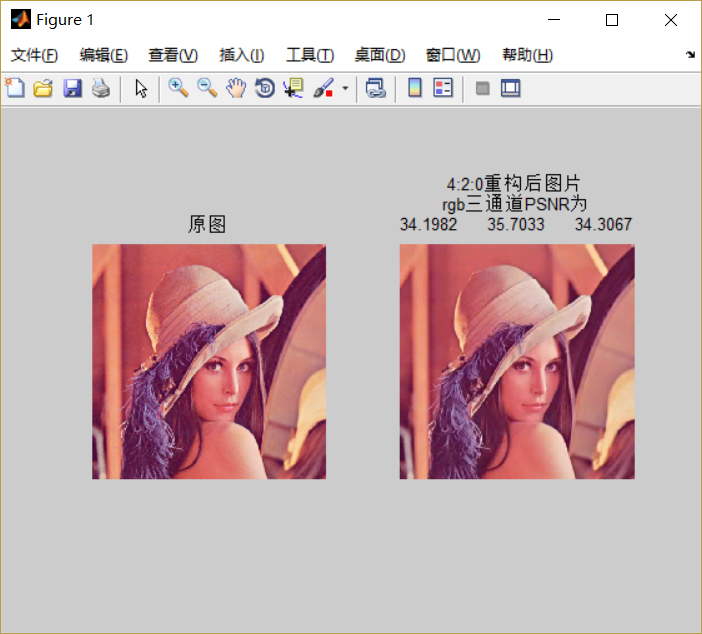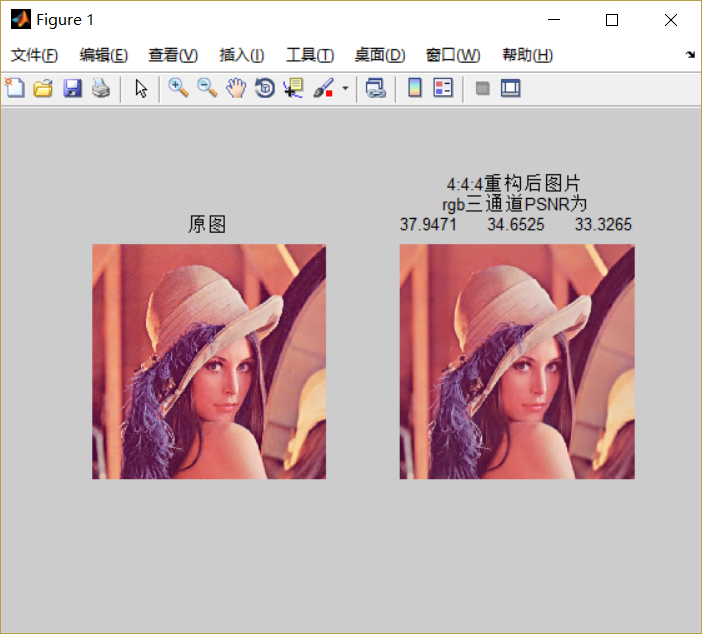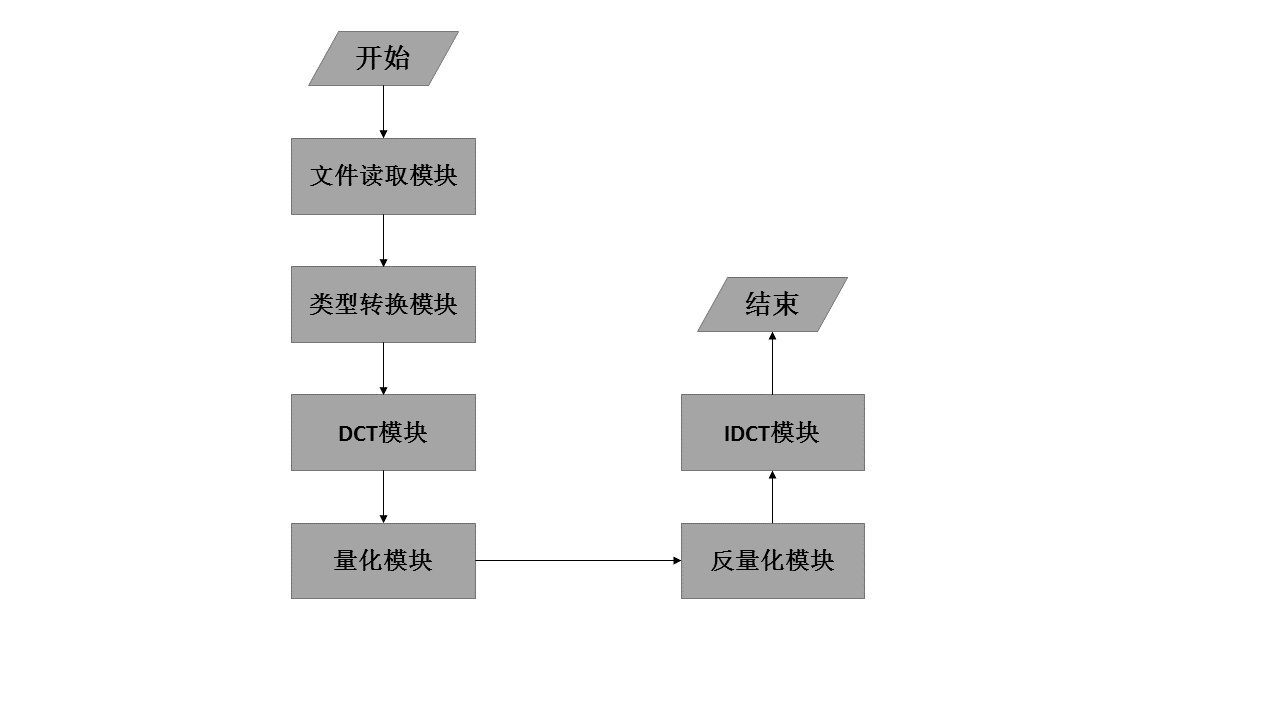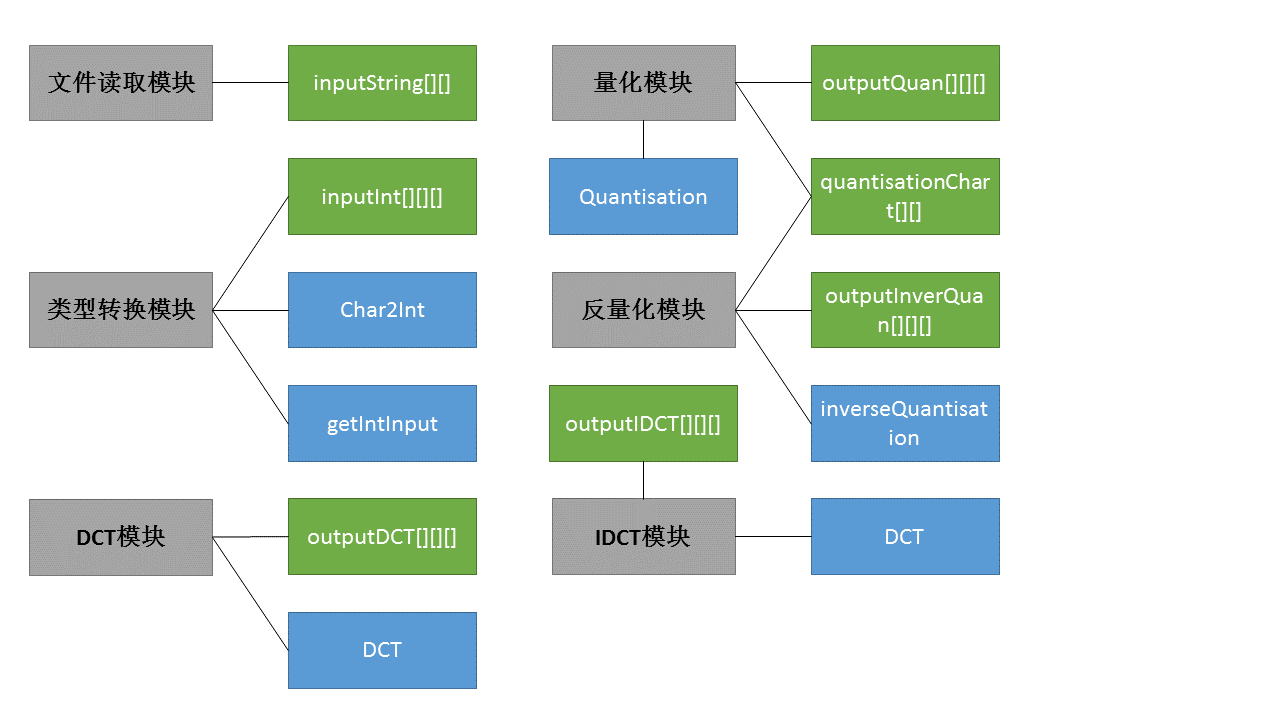• 用于生成jpeg量化表的python程序代码，(依据标准量化表，质量因子从0到100)。
• 使用matlab程序，根据不同的质量因子QF(从60到100)生成对应的标准jpeg亮度与色度量化表
• JPEG标准亮度色度量化表

热门讨论 2012-07-28 19:13:47
IEEE提供的JPEG标准亮度色度量化表(文本形式),共享给需要用到JPEG压缩的程序猿们
• 如果对ycbcr采样不太了解的同学，warp gate：https://blog.csdn.net/xueyushenzhou/article/details/40817949 简单的说，4:2:0就是第一行为y:cb=4:2，没有...%ycbc4:2:0采样压缩 + 量化表ycbc量化压缩 clear all...

如果对ycbcr采样不太了解的同学，warp gate：https://blog.csdn.net/xueyushenzhou/article/details/40817949

简单的说，4:2:0就是第一行为y:cb=4:2，没有cr分量,那么第二行就是y:cr=4:2，没有cb分量。以后每一行一直这样循环。

%ycbcr4:2:0采样压缩 + 量化表ycbc量化压缩

clear all;
img_ycbcr=rgb2ycbcr(f)
[row,col,~]=size(img_ycbcr);

%对Y,Cb,Cr分量进行4:2:0采样
Y(:,:,1)=img_ycbcr(:,:,1);  %Y分量
for i=1:2:row-1
for j=1:2:col-1
Cb((i+1)/2,(j+1)/2)=double(img_ycbcr(i,j,2));
end
end

for i=2:2:row
for j=1:2:col-1
Cr(i/2,(j+1)/2)=double(img_ycbcr(i,j,3));
end
end
Y_Table=[ 16  11  10  16  24  40  51  61 ; ...
12  12  14  19  26  58  60  55 ; ...
14  13  16  24  40  57  69  56 ; ...
14  17  22  29  51  87  80  62 ; ...
18  22  37  56  68 109 103  77 ; ...
24  35  55  64  81 104 113  92 ; ...
49  64  78  87 103 121 120 101 ; ...
72  92  95  98 112 100 103  99 ];%亮度量化表

CbCr_Table=[17  18  24  47  99  99  99  99 ; ...
18  21  26  66  99  99  99  99 ; ...
24  26  56  99  99  99  99  99 ; ...
47  66  99  99  99  99  99  99 ; ...
99  99  99  99  99  99  99  99 ; ...
99  99  99  99  99  99  99  99 ; ...
99  99  99  99  99  99  99  99 ; ...
99  99  99  99  99  99  99  99 ];%色差量化表

%对三个通道分别DCT和量化
Y_dct_q=Dct_Quantize(Y,Y_Table);
Cb_dct_q=Dct_Quantize(Cb,CbCr_Table);
Cr_dct_q=Dct_Quantize(Cr,CbCr_Table);

%对三个通道分别反量化和反DCT
Y_in_q_dct=Inverse_Quantize_Dct(Y_dct_q,Y_Table);
Cb_in_q_dct=Inverse_Quantize_Dct(Cb_dct_q,CbCr_Table);
Cr_in_q_dct=Inverse_Quantize_Dct(Cr_dct_q,CbCr_Table);

%恢复出YCBCR图像
YCbCr_in(:,:,1)=Y_in_q_dct;
for i=1:row/2
for j=1:col/2
YCbCr_in(2*i-1,2*j-1,2)=Cb_in_q_dct(i,j);
YCbCr_in(2*i,2*j-1,2)=Cb_in_q_dct(i,j);
YCbCr_in(2*i-1,2*j,2)=Cb_in_q_dct(i,j);
YCbCr_in(2*i,2*j,2)=Cb_in_q_dct(i,j);
YCbCr_in(2*i-1,2*j-1,3)=Cr_in_q_dct(i,j);
YCbCr_in(2*i,2*j-1,3)=Cr_in_q_dct(i,j);
YCbCr_in(2*i-1,2*j,3)=Cr_in_q_dct(i,j);
YCbCr_in(2*i,2*j,3)=Cr_in_q_dct(i,j);
end
end
image=ycbcr2rgb(YCbCr_in);
MAX=255;
MES=sum(sum((f-image).^2))/(row*col);     %均方差
PSNR=20*log10(MAX/sqrt(MES));             %峰值信噪比
subplot(121),imshow(f);title('原图');
subplot(122),imshow(image);title({'重构后图片';'rgb三通道PSNR为';num2str(PSNR)});

%ycbcr4:4:4采样 + 量化表ycbc量化压缩

clear all;
img_ycbcr=rgb2ycbcr(f)
[row,col,~]=size(img_ycbcr);

Y=img_ycbcr(:,:,1);               %Y分量
Cb=img_ycbcr(:,:,2);
Cr=img_ycbcr(:,:,3);
Y_Table=[16  11  10  16  24  40  51  61;
12  12  14  19  26  58  60  55;
14  13  16  24  40  57  69  56;
14  17  22  29  51  87  80  62;
18  22  37  56  68 109 103  77;
24  35  55  64  81 104 113  92;
49  64  78  87 103 121 120 101;
72  92  95  98 112 100 103  99];%亮度量化表

CbCr_Table=[17, 18, 24, 47, 99, 99, 99, 99;
18, 21, 26, 66, 99, 99, 99, 99;
24, 26, 56, 99, 99, 99, 99, 99;
47, 66, 99 ,99, 99, 99, 99, 99;
99, 99, 99, 99, 99, 99, 99, 99;
99, 99, 99, 99, 99, 99, 99, 99;
99, 99, 99, 99, 99, 99, 99, 99;
99, 99, 99, 99, 99, 99, 99, 99];%色差量化表

%对三个通道分别DCT和量化
Y_dct_q=Dct_Quantize(Y,Y_Table);
Cb_dct_q=Dct_Quantize(Cb,CbCr_Table);
Cr_dct_q=Dct_Quantize(Cr,CbCr_Table);

%对三个通道分别反量化和反DCT
Y_in_q_dct=Inverse_Quantize_Dct(Y_dct_q,Y_Table);
Cb_in_q_dct=Inverse_Quantize_Dct(Cb_dct_q,CbCr_Table);
Cr_in_q_dct=Inverse_Quantize_Dct(Cr_dct_q,CbCr_Table);

%恢复出YCBCR图像
YCbCr_in(:,:,1)=Y_in_q_dct;
YCbCr_in(:,:,2)=Cb_in_q_dct;
YCbCr_in(:,:,3)=Cr_in_q_dct;

I=ycbcr2rgb(YCbCr_in);
MAX=255;
MES=sum(sum((I-f).^2))/(row*col);     %均方差
PSNR=20*log10(MAX/sqrt(MES));             %峰值信噪比
subplot(121),imshow(f);title('原图');
subplot(122),imshow(I);title({'重构后图片';'rgb三通道PSNR为';num2str(PSNR)});

DCT和IDCT函数

function [Matrix]=Dct_Quantize(I,Qua_Table)

I=double(I)-128;   %层次移动128个灰度级
I=blkproc(I,[8 8],'dct2(x)');      %x就是每一个分成的8*8大小的块
Qua_Matrix=Qua_Table;              %量化矩阵
I=blkproc(I,[8 8],'fix(x./P1)',Qua_Matrix);  %量化，四舍五入
Matrix=I;          %得到量化后的矩阵

function [ Matrix ] = Inverse_Quantize_Dct(I,Qua_Table)
Qua_Matrix=Qua_Table;     %反量化矩阵
I=blkproc(I,[8 8],'x.*P1',Qua_Matrix);%反量化，四舍五入
I=blkproc(I,[8 8],'idct2(x)');
I=uint8(I+128);
I(I>255)=255;
I(I<0)=0;
Matrix=I;       %反量化和反Dct后的矩阵

结果：展开全文图像处理 jpeg ycbcr
• JPEG算法解密（三）

千次阅读 2016-08-17 17:31:07
步骤四：数据量化  经过上一节介绍的离散余弦变换，图像数据虽然已经面目全非，但仍然是处于“可逆”的状态，也就是说我们还没有进入“有损”的那一步。这次我们来玩真的，看一下数据中的细节是如何被滤去的。先...

步骤四：数据量化

经过上一节介绍的离散余弦变换，图像数据虽然已经面目全非，但仍然是处于“可逆”的状态，也就是说我们还没有进入“有损”的那一步。这次我们来玩真的，看一下数据中的细节是如何被滤去的。先来考察一下要对付的问题是什么，经过颜色空间转换和离散余弦变换，每一个8X8的图像块都变成了三个8X8的浮点数矩阵，分别表示Y,Cr,Cb数据，比如以其中某个亮度数据矩阵举例，它的数据如下我们的问题是，在可以损失一部分精度的情况下，如何用更少的空间存储这些浮点数？答案是使用量子化（ Quantization ），简称量化。“量子”这个概念来自于物理学，意思是说连续的能量可以看做是一个个单元体的组合，看起来高端大气，其实很简单，比如游戏中在处理角色面朝方向时，一般并不是使用0到2π这样的浮点数，而是把方向分成16个区间，用0到16这样的整数来表示，这样只用4个bit就足够了。JPEG提供的量子化算法如下：(3.1)

其中G是我们需要处理的图像矩阵，Q称作量化系数矩阵（Quantization matrices），JPEG算法提供了两张标准的量化系数矩阵，分别用于处理亮度数据Y和色差数据Cr以及Cb。标准亮度量化表标准色差量化表

其中round函数是取整函数，但考虑到了四舍五入，也就是说(3.2)

比如上面数据，以左上角的-415.38为例，对应的量子化系数是16，那么round(-415.38/16)=round(-25.96125)=-26。最终得到的量子化后的结果为可以看到，一大部分数据变成了0，这非常有利于后面的压缩存储。这两张神奇的量化表也是有讲究的，还记得我们在第一节中所讲的有损压缩的基本原理吗，有损压缩就是把数据中重要的数据和不重要的数据分开，然后分别处理。DCT系数矩阵中的不同位置的值代表了图像数据中不同频率的分量，这两张表中的数据时人们根据人眼对不不同频率的敏感程度的差别所积累下的经验制定的，一般来说人眼对于低频的分量必高频分量更加敏感，所以两张量化系数矩阵左上角的数值明显小于右下角区域。在实际的压缩过程中，还可以根据需要在这些系数的基础上再乘以一个系数，以使更多或更少的数据变成0，我们平时使用的图像处理软件在省城jpg文件时，在控制压缩质量的时候，就是控制的这个系数。
在进入下一节之前，矩阵的量化还有最后一步要做，就是把量化后的二维矩阵转变成一个一维数组，以方便后面的霍夫曼压缩，但在做这个顺序转换时，需要按照一个特定的取值顺序。这么做的目的只有一个，就是尽可能把0放在一起，由于0大部分集中在右下角，所以才去这种由左上角到右下角的顺序，经过这种顺序变换，最终矩阵变成一个整数数组

-26,-3,0,-3,-2,-6,2,-4,1,-3,0,1,5,,1,2,-1,1,-1,2,0,0,0,0,0,-1,-1,0,0,0,0,…,0,0

后面的工作就是对这个数组进行再一次的哈夫曼压缩，已得到最终的压缩数据。
展开全文图像 压缩
• JPEG编码中的DCT与量化

千次阅读 2017-03-16 01:27:51
JPEG是最常用的有损图像压缩算法，而8×8的二维DCT（离散余弦变换）变换和量化又是该算法中的核心步骤。本文作业的例子，是针对一个8×8的数据块进行正反二维DCT变换、量化和反量化

研一上很快过去了，留下了若干课程资料的文件夹。最近整理一下这些资料，挑选一些有用的课程作业贴到博客里面来。

1. 简介

JPEG是最常用的有损图像压缩算法，而8×8的二维DCT（离散余弦变换）变换和量化又是该算法中的核心步骤。本文作业的例子，是针对一个8×8的数据块进行正反二维DCT变换、量化和反量化。

2. 作业题目

逐个读入8×8（十六进制文本串格式的）亮度数据块，依次进行二维DCT变换、量化（采用标准亮度量化表）、逆量化和逆二维DCT变换。输出原始数据、变换后的数据、量化表、量化后的数据、逆量化的数据和反变换的数据。

3. 过程4. 源码

/*
* main.cpp
*
*  Created on: 2016.10.17
*  Author: liboyang
*/
#include <iostream>
#include <stdlib.h>
#include <math.h>
#include <fstream>
#include <string>
#include <iomanip>
#define MAXSIZE 64
#define COL 8
#define ROW 8
#define PI 3.1415926
using namespace std;

int Char2Int(char c);

void getIntInput(int index, int counterRow, string input);

void DCT(int index);

void IDCT(int index);

void Quantisation(int index);

void inverseQuantisation(int index);

string inputString[ROW];
int inputInt[ROW][COL];
double outputDCT[ROW][COL];
double outputIDCT[ROW][COL];
double outputQuan[ROW][COL];
double outputInverseQuan[ROW][COL];
int quantisationChart[ROW][COL]= {
{16,11,10,16,24,40,51,61},
{12,12,14,19,26,58,60,55},
{14,13,16,24,40,57,69,56},
{14,17,22,29,51,87,80,62},
{18,22,37,56,68,109,103,77},
{24,35,55,64,81,104,113,92},
{49,64,78,87,103,121,120,101},
{72,92,95,98,112,100,103,99}};

int main() {
ifstream inFile;

inFile.open("./input.txt", ios::in);

if (NULL == inFile) {
cout << "文件打开失败！" << endl;
return 1;
}
int counterInput = 0, indexInput = 0;
counterInput++;
if (counterInput == 7) {
counterInput = 0;
indexInput++;
counterInput--;
}
}
/*cout << "源图像样本16进制" << endl;
for (int i = 0; i < 4; i++) {
for (int j = 0; j < ROW; j++) {
cout << setw(2) << inputString[i][j] << endl;
}
cout << endl;
}*/

/* Change Hex to Dec */
for (int i = 0; i < 4; i++) {
for (int j = 0; j < ROW; j++) {
getIntInput(i, j, inputString[i][j]);
}
}

cout << endl << "源图像样本10进制" << endl;
for (int m = 0; m < 4; m++) {
for (int i = 0; i < ROW; i++) {
for (int j = 0; j < COL; j++) {
cout << setw(2) << inputInt[m][i][j] << " \t";
}
cout << endl;
}
cout << endl;
}

cout << endl << "DCT" << endl;
/* DCT */
/* Each element minus 128 */
for (int m = 0; m < 4; m++) {
for (int i = 0; i < ROW; i++) {
for (int j = 0; j < COL; j++) {
inputInt[m][i][j] -= 128;
}
}
}
for (int i = 0; i < 4; i++) {
DCT(i);
}
for (int m = 0; m < 4; m++) {
for (int i = 0; i < ROW; i++) {
for (int j = 0; j < COL; j++) {
cout << setw(2) << fixed << setprecision(1) << outputDCT[m][i][j] << " \t";
}
cout << endl;
}
cout << endl;
}

cout << endl << "标准量化表" << endl;
/* Quantisation */
for (int i = 0; i < ROW; i++) {
for (int j = 0; j < COL; j++) {
cout << setw(2) << quantisationChart[i][j] << " \t";
}
cout << endl;
}

for (int i = 0; i < 4; i++) {
Quantisation(i);
}
cout << endl << "量化系数" << endl;
for (int m = 0; m < 4; m++) {
for (int i = 0; i < ROW; i++) {
for (int j = 0; j < COL; j++) {
cout << setw(2) << fixed << setprecision(0) << outputQuan[m][i][j] << " \t";
}
cout << endl;
}
cout << endl;
}

for (int i = 0; i < 4; i++) {
inverseQuantisation(i);
}
cout << endl << "反量化系数" << endl;
for (int m = 0; m < 4; m++) {
for (int i = 0; i < ROW; i++) {
for (int j = 0; j < COL; j++) {
cout << setw(2) << fixed << setprecision(0) << outputInverseQuan[m][i][j] << " \t";
}
cout << endl;
}
cout << endl;
}

cout << endl << "IDCT" << endl;
for (int i = 0; i < 4; i++) {
IDCT(i);
}
/* Each element add 128 */
for (int m = 0; m < 4; m++) {
for (int i = 0; i < ROW; i++) {
for (int j = 0; j < COL; j++) {
outputIDCT[m][i][j] += 128;
}
}
}
for (int m = 0; m < 4; m++) {
for (int i = 0; i < ROW; i++) {
for (int j = 0; j < COL; j++) {
cout << setw(2) << fixed << setprecision(0) << outputIDCT[m][i][j] << " \t";
}
cout << endl;
}
cout << endl;
}
system("pause");
return 0;
}

int Char2Int(char c) {
if (c >= '0'&& c <= '9') {
return c - '0';
}
else if (c >= 'A' && c <= 'F') {
return c - 'A' + 10;
}
else if (c >= 'a' && c <= 'f') {
return c - 'a' + 10;
}
return -1;
}

void getIntInput(int index, int counterRow, string input) {
int first, second;
int counter = 0;
for (int i = 0; i < MAXSIZE; i+=3) {
first = Char2Int(input[i]);
second = Char2Int(input[i+1]);
if (first != -1 && second != -1) {
inputInt[index][counterRow][counter] = second + first * 16;
counter++;
}
}
}

void DCT(int index) {
double ALPHA, BETA;
int u = 0;
int v = 0;
int i = 0;
int j = 0;
for (u = 0; u < ROW; u++) {
for (v = 0; v < COL; v++) {
if (u == 0) {
ALPHA = 1.0 / sqrt(2);
}
else {
ALPHA = 1.0;
}
if (v == 0) {
BETA = 1.0 / sqrt(2);
}
else {
BETA = 1.0;
}
double tmp = 0.0;
for (i = 0; i < ROW; i++) {
for (j = 0; j < COL; j++) {
tmp += inputInt[index][i][j] * cos((2*i+1)*u*PI/16) * cos((2*j+1)*v*PI/16);
}
}
outputDCT[index][u][v] = ALPHA * BETA * tmp * 0.25;
}
}
}

void Quantisation(int index) {
int u, v;
for (u = 0; u < ROW; u++) {
for (v = 0; v < COL; v++) {
outputQuan[index][u][v] = round(outputDCT[index][u][v] / quantisationChart[u][v]);
if (outputQuan[index][u][v] == -0) {
outputQuan[index][u][v] = 0;
}
}
}
}

void inverseQuantisation(int index) {
int u, v;
for (u = 0; u < ROW; u++) {
for (v = 0; v < COL; v++) {
outputInverseQuan[index][u][v] = outputQuan[index][u][v] * quantisationChart[u][v];
if (outputInverseQuan[index][u][v] == -0) {
outputInverseQuan[index][u][v] = 0;
}
}
}
}

void IDCT(int index) {
double ALPHA, BETA;
int u = 0;
int v = 0;
int i = 0;
int j = 0;
for (i = 0; i < ROW; i++) {
for (j = 0; j < COL; j++) {
double tmp = 0.0;
for (u = 0; u < ROW; u++) {
for (v = 0; v < COL; v++) {
if (u == 0) {
ALPHA = 1.0 / sqrt(2);
}
else {
ALPHA = 1.0;
}
if (v == 0) {
BETA = 1.0 / sqrt(2);
}
else {
BETA = 1.0;
}
tmp += ALPHA * BETA * cos((2*i+1)*u*PI/16) * cos((2*j+1)*v*PI/16) * outputInverseQuan[index][u][v];
}
}
outputIDCT[index][i][j] = 0.25 * tmp;
}
}
}

展开全文JPEG 编码
• 将任意原始图像经过jpeg压缩，是一个有损压缩过程，信息量的损失是按照量化矩阵对DCT系数进行round到整数量化时出现的，不同的量化矩阵会导致不同的jpeg压缩率。随着压缩率的增加相应的图像清晰度也会变低，具体压缩...

jpeg压缩过程

1. 将任意原始图像经过jpeg压缩，是一个有损压缩过程，信息量的损失是按照量化矩阵对DCT系数进行round到整数量化时出现的，不同的量化矩阵会导致不同的jpeg压缩率。随着压缩率的增加相应的图像清晰度也会变低，具体压缩率与量化矩阵的关系见此链接

2. 压缩率由品质因数（Q）反应，Q的取值范围从1-100，取1时图像压缩率最高，最不清晰，量化矩阵的所有元素值均为255；取100时图像没有压缩，与原图相同，量化矩阵的所有元素值都为1；当Q取50时，就是现有博客中常见的量化矩阵系数：3. 量化矩阵作用在YUV通道经过DCT处理后的8*8矩阵上，同时需要注意的是量化矩阵有两种，一种为亮度量化矩阵，作用在Y通道，一种为色度量化矩阵，作用在U、V通道。

用MATLAB生成任意压缩率的jpeg图片

imwrite(Ireference,fullfile('下载Quality10.jpg'),'Quality',10);
imwrite(Ireference,fullfile('下载Quality50.jpg'),'Quality',50);
imwrite(Ireference,fullfile('下载Quality100.jpg'),'Quality',100);
imwrite(Ireference,fullfile('下载Quality1.jpg'),'Quality',1);
imwrite(Ireference,fullfile('下载Quality60.jpg'),'Quality',60);

montage({I1,I10,I50,I60,I100,Ireference},'Size',[2 3])
title('JPEG-Compressed Images with Quality Factor: 1,10,50,60,100 and origin (left to right,up to down)')可以直观对比不同Quality Factor下的图片的视觉效果以及占用内存情况。

利用C语言提取已有jpeg文件的量化矩阵(quantization matrix)

需要安装JpegLib库
JpegLib库下载、安装及使用
之后需要在visual studio 2015中包括 libjpeg.lib
然后写代码在visual studio 2015中处理上述在MATLAB中生成的不同压缩率的图片，以品质因数Q的值为50作为示例

#include <stdio.h>
#include <stdlib.h>
#include <string.h>
#include <string>
#include <iostream>
using namespace std;

extern "C" {
#include "jpeglib.h"
}

#pragma comment(lib, "libjpeg.lib")

int main(int argc, char **argv)
{
FILE *file;
char fileName;

int width;
int height;
struct jpeg_decompress_struct jDecompressStruct;
struct jpeg_error_mgr jErrorMgr;

int i = 50;
//表示品质因数为50，处理"下载Quality50.jpg"这个文件
//当想处理品质因数为1或10或60或100的文件时，相应修改i的值即可
{
memset(fileName, 0x00, sizeof(fileName));

strcat(fileName, "D:\\你在MATLAB中生成图片的路径\\下载Quality");
//这里需要改成你自己的路径，但"\\下载Quality"不要改变

char jpjnum;
itoa(i, jpjnum, 10); //方便进行批处理 数字转为字符串
char * Extend;
Extend = ".jpg";
strcat(jpjnum, Extend);
strcat(fileName, jpjnum);

if (strcmp(fileName, "q") == 0)
{
exit(0);
}
if ((file = fopen(fileName, "rb")) == 0) {
fprintf(stderr, "can't open %s\n", fileName);
}

// 初始化并申请解码器
jDecompressStruct.err = jpeg_std_error(&jErrorMgr);
jpeg_create_decompress(&jDecompressStruct);

// 指定图片文件信息
jpeg_stdio_src(&jDecompressStruct, file);

// 读取头部信息

// 开始解码
jpeg_start_decompress(&jDecompressStruct);

// 获取图片宽高
width = jDecompressStruct.image_width;
height = jDecompressStruct.image_height;

auto thisva = jDecompressStruct.quant_tbl_ptrs->quantval;
cout << thisva << endl;
//输出亮度量化矩阵的第一个元素

printf("decompress file:%s, %d x %d\n", fileName, width, height);

// 释放解码器对象
jpeg_destroy_decompress(&jDecompressStruct);

fclose(file);

}
return 0;
}

将断点加在下述图片所示位置进行调试在变量区中，进入jDecompressStruct变量，找到quant_tbl_ptrs变量，可以看到quant_tbl_ptrs有四个值，索引处的数值序列表示亮度量化矩阵，索引处的数值序列表示色度量化矩阵。展开来看，有如下数值:将这些数值与当Q取50时现有博客中常见的量化矩阵进行对比，发现完全一样。
进而得到了从jpg文件中获取亮度（luminance matrix）、色度（chroma matrix）量化矩阵信息的方法。
进一步地，可以修改i为1、10、60、100，通过调试程序在变量区查看对应的量化矩阵的变化。

展开全文matlab 计算机视觉 c语言 算法
• JPEG压缩算法

2020-02-27 22:21:00
JPEG算法解密  by jinchao             图片压缩有多重要，可能很多人可能并没有一个直观上的认识，举个例子，一张800X800大小的普通图片...
• JPEG 标准推荐的亮度、色度DC、AC Huffman 编码 博主在完成数字图像处理大作业时利用搜索引擎查找了很久完整的四张Huffman 编码(亮度AC Huffman编码亮度DC Huffman编码、色度AC Huffman编码、色度DC ...图像处理
• JPEG 原理详细分析

千次阅读 2020-11-18 16:43:32
JPEG 概述 JPEG 是 Joint Photographic Experts Group 的缩写，即 ISO 和 IEC 联合图像专家组，负责静态图像压缩标准的制定，这个专家组开发的算法就被称为 JPEG 算法，并且已经成为了大家通用的标准，即 JPEG ...
• JPEG 编码器 离散余弦变换，图像数据虽然已经面目全非，但仍然是处于“可逆”的状态，也就是说我们还没有进入“有损”的那一步。这次我们来玩真的，看一下数据中的细节是如何被滤去的。先来考察一下要对付的问题...矩阵
• 通过量化可以reducing the number of bits and eliminating some of the components，达到通低频减高频的效果，如下图所示就是两张量化表的例子： 比如左边那个量化表，最右下角的高频÷99，这样原先DCT后[-127,127...
• 读取JPEG头文件中的huffman表

千次阅读 2018-12-27 16:00:10
读取JPEG头文件中的huffman 实验工具：UltrEdit、Matlab 实验目的：提取出JPEG头文件中的huffman段，并构建huffman映射。 在讲实验前，先了解一下JPEG的格式。 一.JPEG格式 JPEG格式是一种常见的图像文件...Matlab JPEG头文件 huffman表
• 根据最小可觉差模型，以图像的局部纹理和局部亮度为参数，将图像压缩过程中的宏块分为6类，对每类宏块的直流、低频、高频系数赋予不同的量化参数，从而使得整幅图像的码率根据纹理复杂度和亮度合理分布，在保持主观...
• 一、jpeg压缩流程的简单介绍 jpeg压缩是基于YUV颜色空间进行压缩编码的，首先将RGB转化成YUV，然后将像素值减去128，将像素值转化到范围-128~127。后要进行采样，一般来说有3中采样方式：4:4:4，4:2:2和4:1:1；4:4:4...
• JPEG压缩原理与DCT离散余弦变换2016年06月20日 15:58:47阅读数：19214原文网址：http://blog.csdn.net/newchenxf/article/details/51719597 转载请注明出处喔1 前言JPEG是joint Photographic Experts Group(联合...
• 文章目录JPEG解码实验一、实验原理（JPEG文件格式）JPEG编码原理1、Segment的组织形式2、DQT（定义量化表）3、SOF04、DHT（定义Huffman树表）5、SOS二、实验步骤1、程序设计整体框架2、结构体设计（1）struct ...
• jpeg编码学习笔记

千次阅读 2016-12-24 21:12:00
各种图片格式目的是在网络传输和存储的时候使用更少的字节，即起到压缩的作用。在图片格式解码后，无论图片的格式，图片数据都是像素数组。...本文将尝试通过JPEG这种图片编码格式的学习，了解图片编码的秘密。编码 压缩
• JPEG编解码原理 图像分块：由于后面的DCT变换是是对8x8的子块进行处理的，因此，必须先将原始图像数据分成8*8的小块。 零偏置（Level Offset）：将所有像素的电平减去128，电平范围从[0,255]变成[-128,127] 8×8 ...
• JPEG 标准推荐的亮度、色度DC、AC Huffman 编码 博主在完成数字图像处理大作业时利用搜索引擎查找了很久完整的四张Huffman 编码(亮度AC Huffman编码亮度DC Huffman编码、色度AC Huffman编码、色度DC ...
• Quantization Tables ...In JPEG, each F[u,v] is divided by a constant q(u,v). Table of q(u,v) is called quantization table. ---------------------------------- 16 11 10 16 24 40 51 61numbers less each
• JPEG标准DCT算法

千次阅读 2013-03-13 18:10:34
JPEG标准   动画演示> 作业>  JPEG(Joint Photographic Experts Group)是联合图像专家小组的英文缩写。其中“联合”的含意是指，国际电报电话咨询委员会(CCITI)和国际标准化协会(ISO)联合组成的一个图像...
• Matlab实现RGB图像DCT量化编码

热门讨论 2013-04-20 11:48:01
用Matlab实现彩色图像的DCT量化，采用标准的JPEG亮度图像量化表进行量化，把RGB分层到R、G、B三层处理，进行8*8分块处理，之后得到使用cat得到重构的RGB图像。DCT编码
• 两张量化表——亮度，色度 四张Huffman码表——亮度DC，亮度AC，色度DC，色度AC 2.1 Level Offset 零偏置 对于灰度级是 2n 的像素，通过减去 2n-1 ，将无符号的整数值变成有符号数。 例如：n=8，灰度级0 ~ 255，...
• 图像编码算法都有相应的质量参数，如hevc编码中的qp值（值越大，压缩率越高），jpeg中的quality（对应到DCT变换后的量化程度）。最近看了看如何根据jpeg图像中的量化文件统计其quality参数，记录下过程。 我的环境：...jpeg 量化表
• JPEG压缩原理详解

千次阅读 多人点赞 2019-03-25 15:36:17
举个例子，一张800X800大小的普通图片，如果未经压缩，大概在1.7MB左右，这个体积如果存放文本文件的话足够保存一部92万字的鸿篇巨著《红楼梦》，现如今互联网上绝大部分图片都使用了JPEG压缩技术，也就是大家使用.....jpeg 压缩
• JPEG是joint Photographic Experts Group(联合图像专家组)的缩写，文件后辍名为”．jpg”或”．jpeg”。 jpg图片可以说是最常见的图片格式了，基本上你的自拍照，要么是png的，要么就是jpeg的了。（有关jpeg和png的...
• 作为一个基本的图像压缩方式，JPEG 已经得到了广泛的运用，但 JPEG 相关的基本原理，却经常被忽视，或解释得很不确切。这里我们详细讨论一下 JPEG 的编码原理，并结合实例来给出一个更加感性的认识。JPEG 编码的详细...
• H.264，H.265视频量化亮度和色度信号QP值选取不同的原因 亮度和色度信号QP值选取不同的原因：对于色差信号，若果采用较大的量化步长会产生颜色漂移现象。因此，当qp值比较小的时候，二者对应相同，当qp值比较大的...H.265 图像压缩...